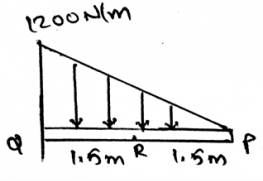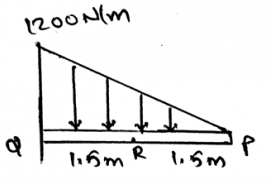# Test: Shear Stress Diagram

## 15 Questions MCQ Test Engineering Mechanics | Test: Shear Stress Diagram

Description
This mock test of Test: Shear Stress Diagram for Mechanical Engineering helps you for every Mechanical Engineering entrance exam. This contains 15 Multiple Choice Questions for Mechanical Engineering Test: Shear Stress Diagram (mcq) to study with solutions a complete question bank. The solved questions answers in this Test: Shear Stress Diagram quiz give you a good mix of easy questions and tough questions. Mechanical Engineering students definitely take this Test: Shear Stress Diagram exercise for a better result in the exam. You can find other Test: Shear Stress Diagram extra questions, long questions & short questions for Mechanical Engineering on EduRev as well by searching above.
QUESTION: 1

### The determination of the internal loading in the beams for shear stress diagrams is usually done so as to:

Solution:

The determination of the internal forces in the beam is done so as to design the beams as in the application purpose the beams will be subjected to many loads. This will help us to make the beam properly. And also this will ensure that the beams will not break after the loading is done on them. For this another method is the distribution load method.

QUESTION: 2

### The change in moment in shear stress diagrams is equal to _________

Solution:

After the application of the force equation of equilibrium to the segment of the beam, we have the above result. This is done on the very small part of the beam. That is the minimal section of the beam is to be considered and then the application of the equilibrium equations are done so as to calculate the final result.

QUESTION: 3

### Determine the shear force of the beam shown.Solution:

The loading acting upward is considered to be positive. This is done as to decrease the time used for the calculations. If the method of sections is being applied at the complex sections for the determination of the unknown forces, much time is required for it. Thus the main motto is to make the calculations easy and make the less use of time.

QUESTION: 4

The couple moment and the force in shear stress diagrams is divided to get the distance of the axis from the point of action of the force.

Solution:

The couple is the cross product of the force and the perpendicular distance between the forces. And if the division of the both is done than the resulting quantity is the distance only. But care must be taken that the product is the cross product and hence sine angle must be divided too to get the distance.

QUESTION: 5

The slope of the shear diagram is equal to__________

Solution:

After the application of the force equation of equilibrium to the segment of the beam, we have the above result. This is done on the very small part of the beam. That is the minimal section of the beam is to be considered and then the application of the equilibrium equations are done so as to calculate the final result.

QUESTION: 6

The equation of change in moment in shear stress diagrams equals area under shear diagram is not applied when?

Solution:

As this is done with the help of distributed load method thus when a concentrated load is applied this equation is not valid. This is done on the very small part of the beam. That is the minimal section of the beam is to be considered and then the application of the equilibrium equations are done so as to calculate the final result.

QUESTION: 7

Change in the shear in shear stress diagrams is equal to ____________

Solution:

After the application of the force equation of equilibrium to the segment of the beam, we have the above result. This is done on the very small part of the beam. That is the minimal section of the beam is to be considered and then the application of the equilibrium equations are done so as to calculate the final result.

QUESTION: 8

Determine the normal force of the beam shown.Solution:

The loading acting upward is considered to be positive. This is done as to decrease the time used for the calculations. If the method of sections is being applied at the complex sections for the determination of the unknown forces, much time is required for it. Thus the main motto is to make the calculations easy and make the less use of time.

QUESTION: 9

The equation of change in moment in shear stress diagrams equals area under shear diagram is not applied when?

Solution:

As this is done with the help of distributed load method thus when a couple moment this equation act is not valid. This is done on the very small part of the beam. That is the minimal section of the beam is to be considered and then the application of the equilibrium equations are done so as to calculate the final result.

QUESTION: 10

The couple in the beam is simplified easily by the help of right hand rule in shear stress diagrams. But the forces simplification is not possible as there is no such system of rules so that the forces can be simplified.

Solution:

Both of them are vector quantities. And both of them can be easily simplified. If taken in the vector form then the task is even easier. Thus it is not necessary for the force or the couple to be vector only, even if the magnitude is taken, the simplification is done in the 2D. Forces are simplified on the basis of the algebra.

QUESTION: 11

Slope of the moment diagram is equal to _________

Solution:

After the application of the force equation of equilibrium to the segment of the beam, we have the above result. This is done on the very small part of the beam. That is the minimal section of the beam is to be considered and then the application of the equilibrium equations are done so as to calculate the final result.

QUESTION: 12

The internal loading in shear stress diagrams can be found by ___________

Solution:

The determination of the internal forces is done by various methods among which the distribution of load method is the one. Also we have method of joints, which is done so as to determine the internal forces which are being developed in the trusses or the frames. This is done as to design them. In this method the loading acting upward is considered to be positive.

QUESTION: 13

In the making of the shear force diagram or the bending moment diagrams, one method is used, distributed load. In this the distributed load will be considered positive if?

Solution:

The loading acting upward is considered to be positive. This is done as to decrease the time used for the calculations. If the method of sections is being applied at the complex sections for the determination of the unknown forces, much time is required for it. Thus the main motto is to make the calculations easy and make the less use of time.

QUESTION: 14

The shear diagram will jump downward if _________

Solution:

After the application of the force equation of equilibrium to the segment of the beam, we have the above result. This is done on the very small part of the beam. That is the minimal section of the beam is to be considered and then the application of the equilibrium equations are done so as to calculate the final result.

QUESTION: 15

The shear diagram will jump upwards if_____________

Solution:

After the application of the force equation of equilibrium to the segment of the beam, we have the above result. This is done on the very small part of the beam. That is the minimal section of the beam is to be considered and then the application of the equilibrium equations are done so as to calculate the final result.